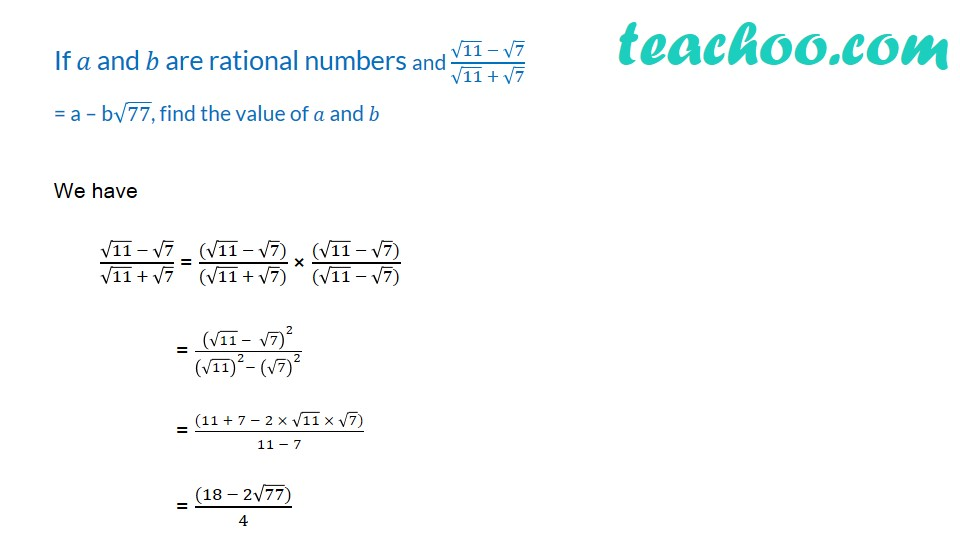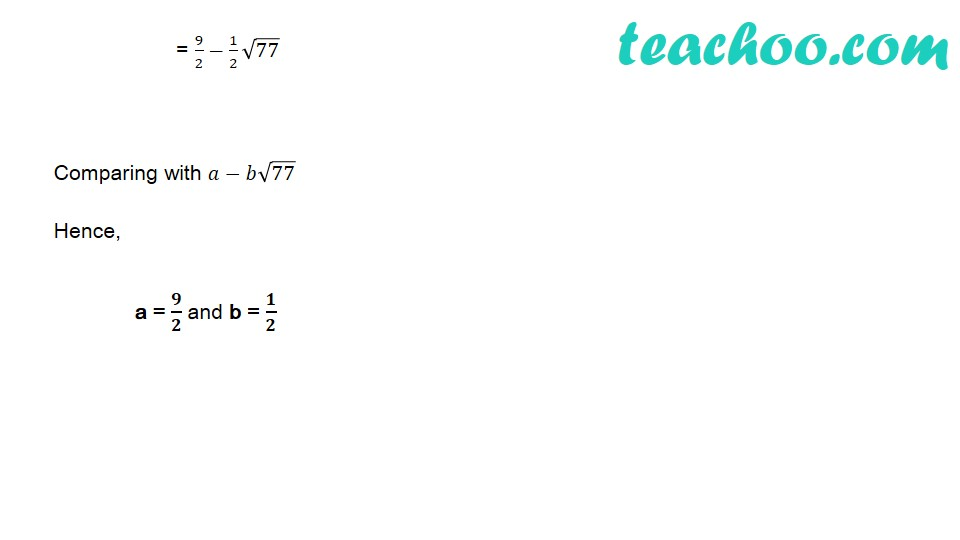1. Chapter 1 Class 9 Number Systems (Term 1)
2. Concept wise
3. Rationalising

Transcript

If 𝑎 and 𝑏 are rational numbers and (√11 − √7)/(√11 + √7) = a – b√77, find the value of 𝑎 and 𝑏 = 9/2−1/2 √77 Comparing with 𝑎−𝑏√77 Hence, a = 𝟗/𝟐 and b = 𝟏/𝟐

Rationalising

Chapter 1 Class 9 Number Systems (Term 1)
Concept wise